## Common Baseline Graph With Parameter (Part 2)

by Maleeha Ahmed

In the first step, I have shown how to create a common baseline graph.

Here I will show you how to use parameters to make this graph more dynamic.

We will use a parameter control to highlight a chosen subcategory and create a dual axis which will create a large highlighted circle that shows the running total number at the end of the timeline for that particular product.

Picking up from where we left off in part One, we have already created our common baseline chart.

Now we will create our parameter control.

Within this configuration box we will:

• Use a String, data type
• List of allowable values
• Populate our list by adding from field – our subcategory list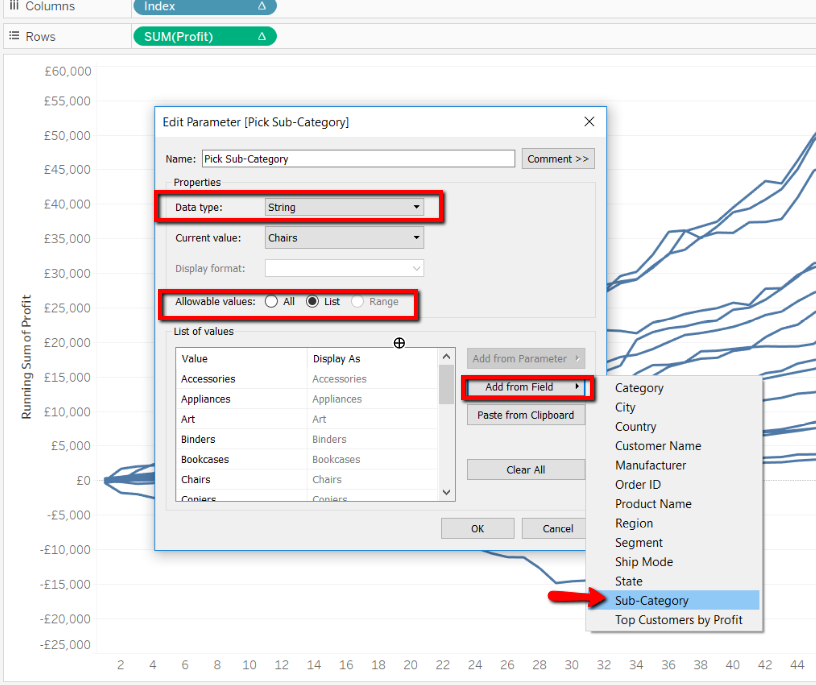Now we will create a calculated field to join our parameter to the data.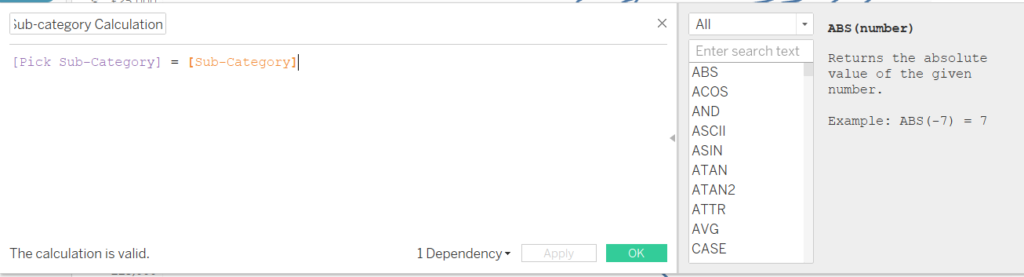Within the calculation, purple means it’s a parameter and orange means it’s a data field.

Here we are creating a Boolean calculation; a true or false statement.

In this calculation, we are saying if parameter equals subcategory its true, else false.

Now let’s show our parameter control. Right click on your parameter and choose show parameter control. The parameter control will show up on the right side of your screen.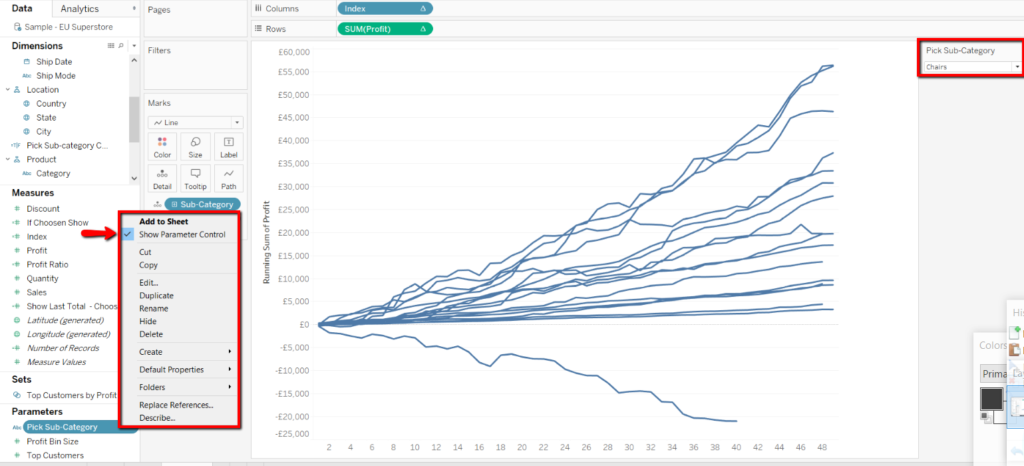Now add our parameter calculation to colour.

Now we have the first step done. We now want to create the dual axis to show the circle at the end of the line that contains the total amount over time.

We will now need to create two more calculated fields.

1st Calculation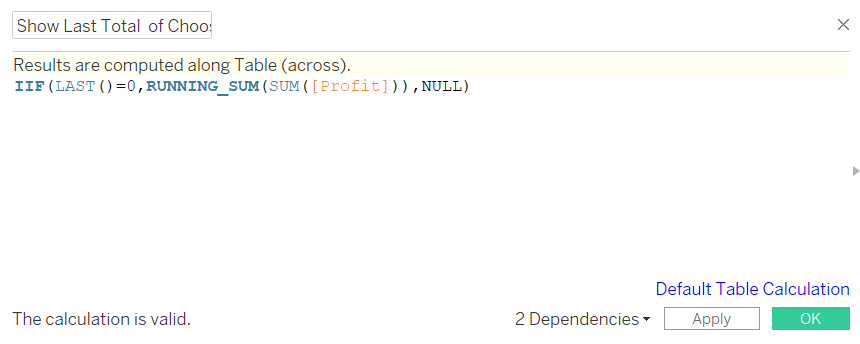This calculation will be used to pin point the last index of time in our graph. When a subcategory is chosen, only the end of that subcategories line will have a circle.

This calculation is saying if the last index equals 0 then return me the running sum of profit, if it is not the last index then give me nothing.

Now we will join this calculation to our parameter so that the parameter will dictate which sub-category shows the last index.

2nd calculation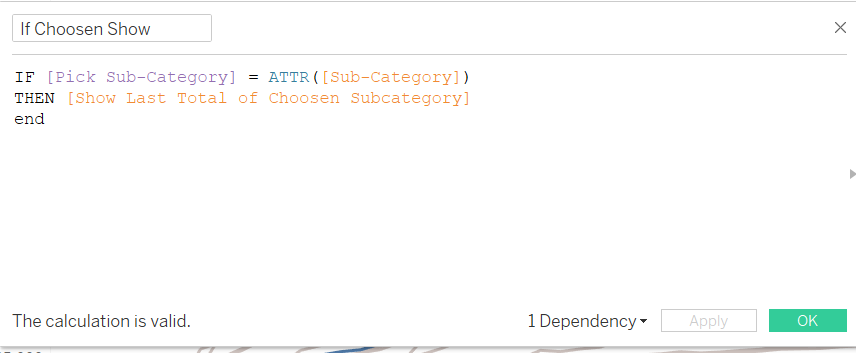In this calculation we are creating that Boolean calculation (true/false) like we did previously. Adding the calculation we have just created to state, when [Pick Sub-Category] = [Sub-Category] is true, then give us our [Show Last Total of Chosen Subcategory] calculation.

Now we will place this new calculation onto the rows shelf and create a dual axis.

What you see now is a line graph of the chosen sub-category on another axis. This is not what we want.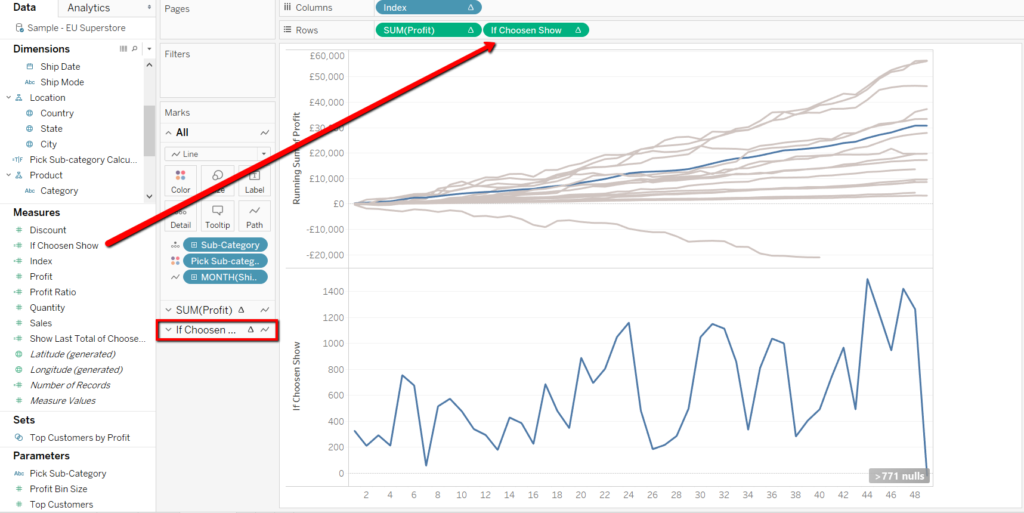We will now need to right click on to our calculation in the rows shelf and edit the table calculation.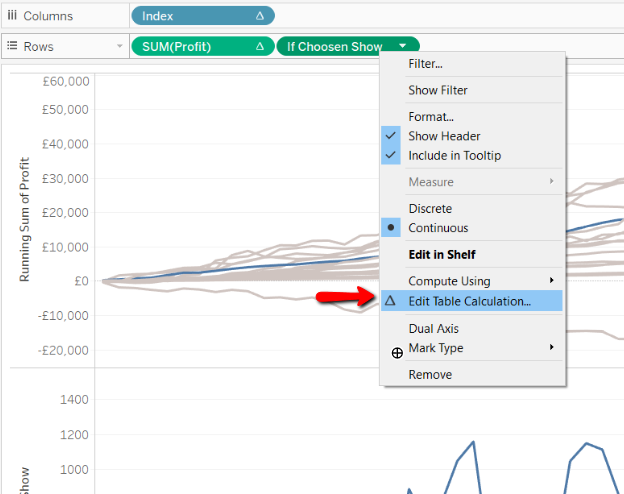Within the configuration window, we always want to choose specific dimensions and we will choose to tick our date field.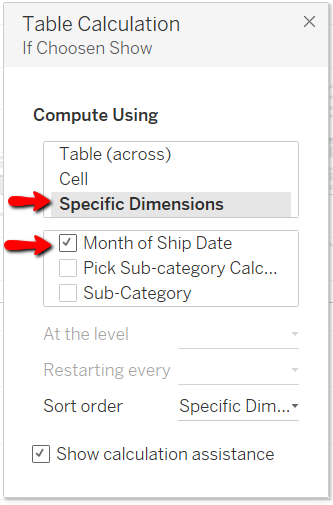Now we will have the single end Circle that we wanted.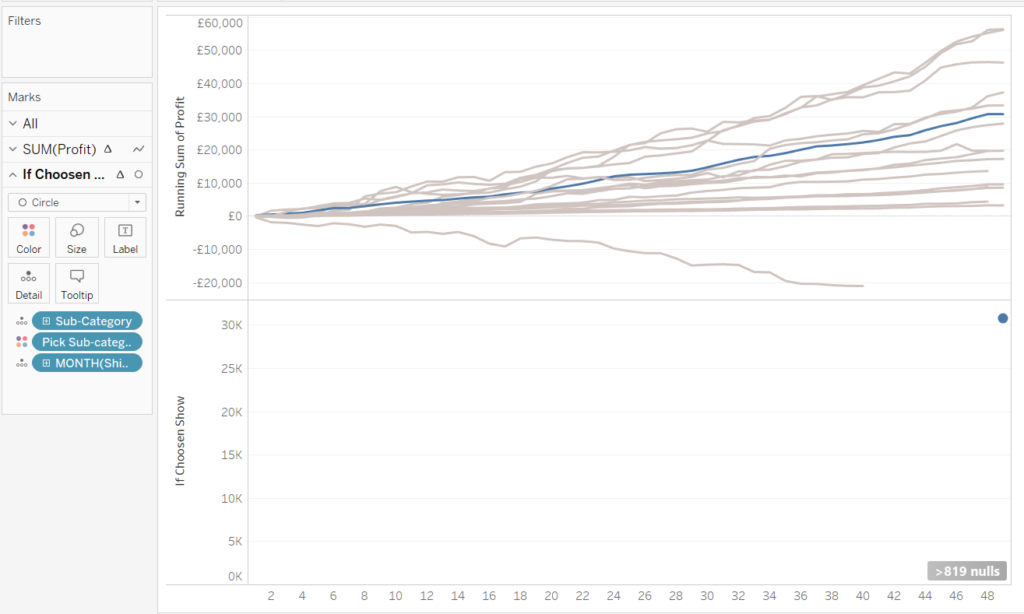Now let’s dual-axis our graph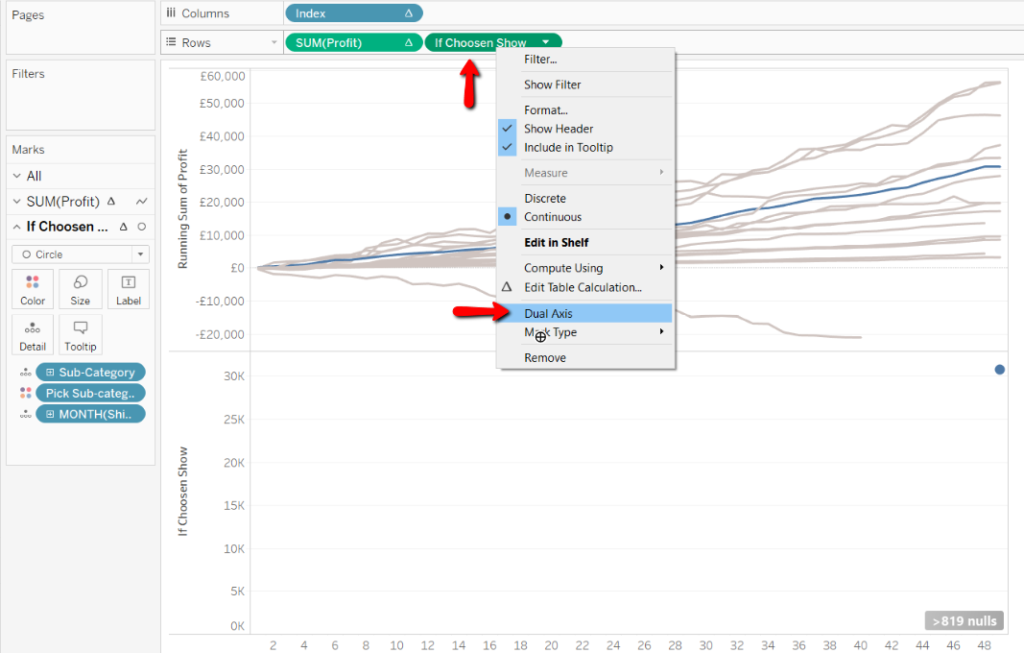Now we need to synchronise our axis. By right clicking our right axis, we can then have the option to select synchronise axis.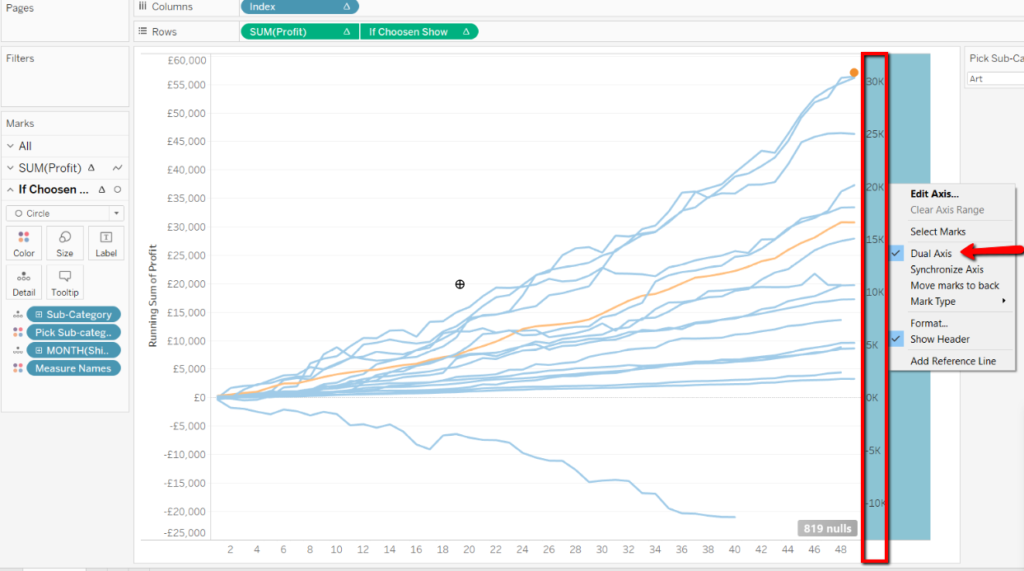Now to get the running total sum number within the circle. Right click and drag our dual “If Chosen Show” calculation on to the text shelf. Make sure you are on the correct shelf when you do this i.e. the shelf which contains the circle.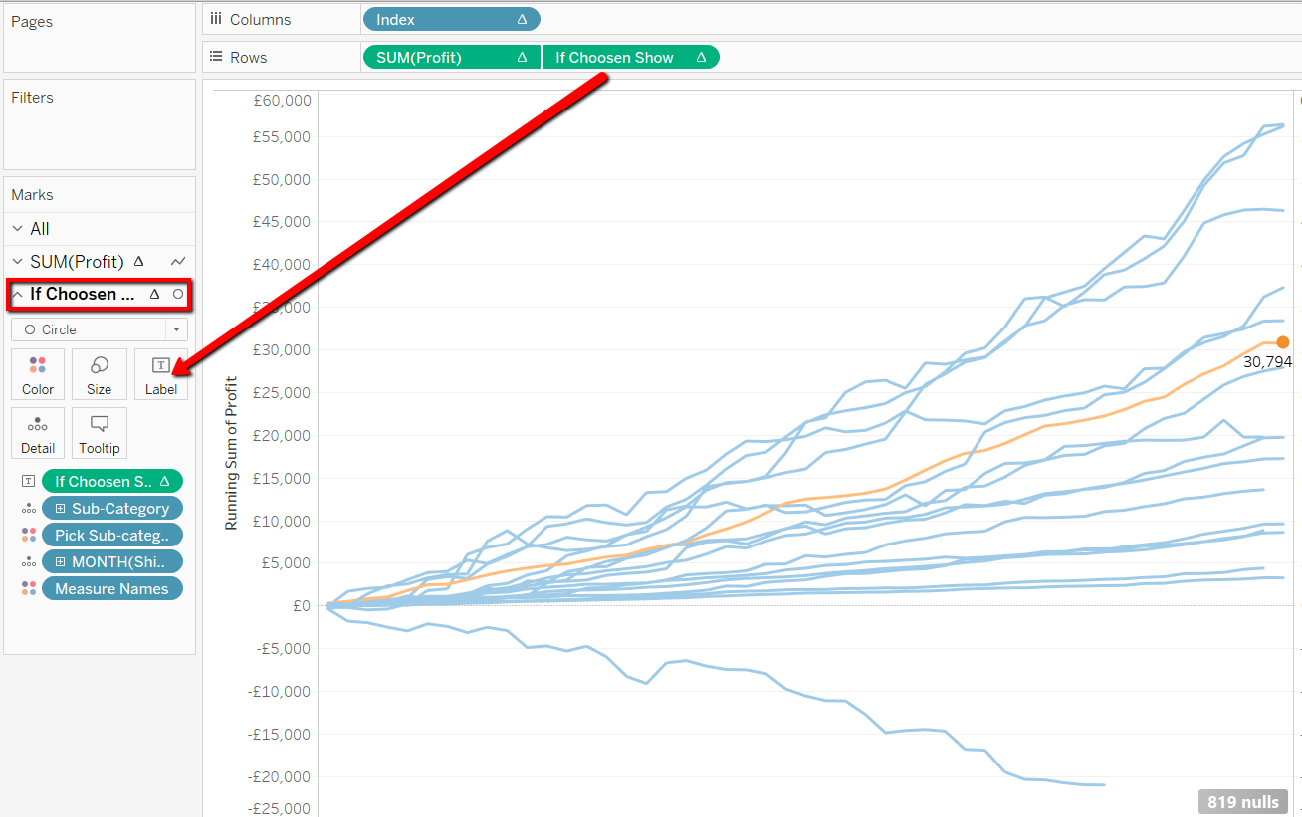We will right click on the calculation and choose format.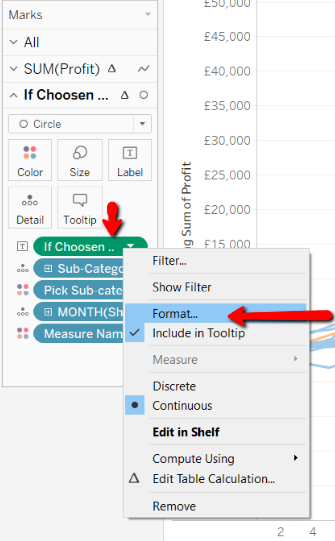I will choose to format this number into a customer currency and rounded to One decimal place.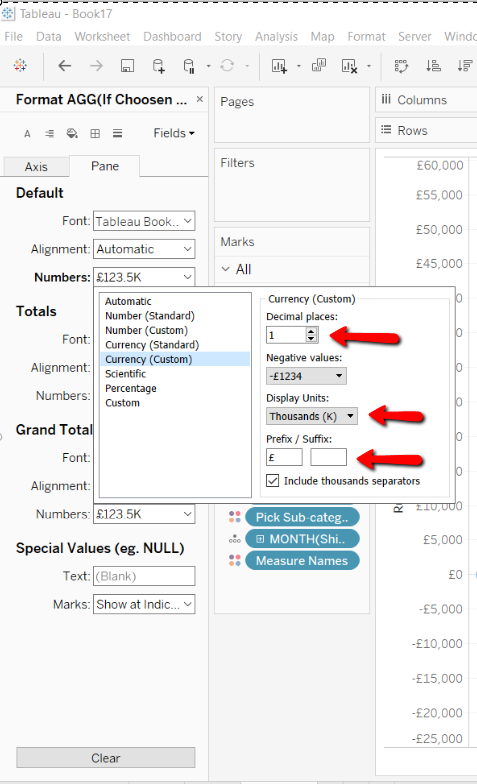Now you can readjust the size of your circle to fit the text inside. Another tip is to change the index into a continuous measure.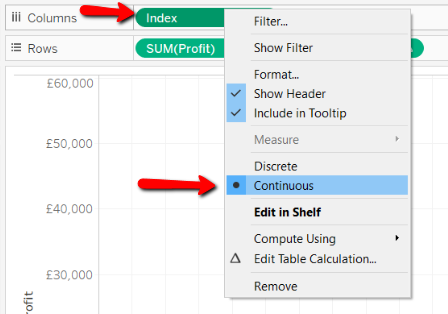On both axis shelves, there will now be an added “Measure Names” on to colour. Remove this off both the shelves.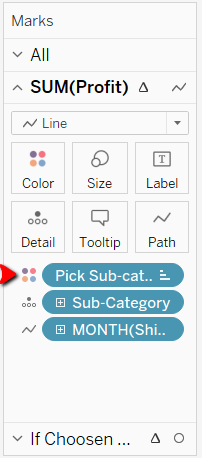You should now only have your pick sub-category calculation on the colour as we had in the beginning.

Now you should have a graph that looks like this.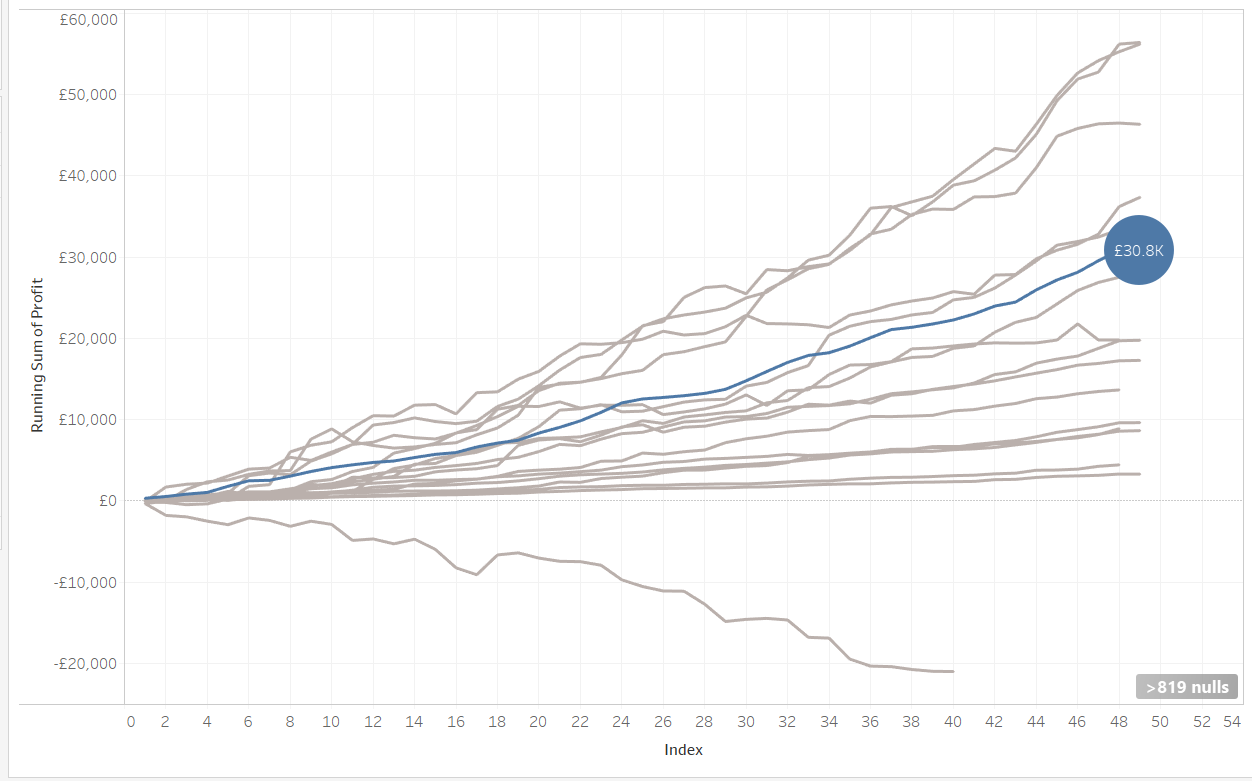Maleeha Ahmed

Fri 01 Feb 2019

Fri 01 Feb 2019

Wed 19 Dec 2018